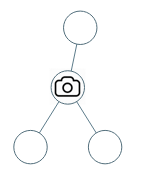# 1、输入：[0,0,null,0,0]输入：[0,0,null,0,null,0,null,null,0]



## 1.1、解题思路

1、 下一层如果能被观测到但没有摄像头，那么本层肯定是不被观测得到的（0->1）；

2、 如果下一层安装了摄像头，本层能够被观测到（2->0）；

3、 下一层如果不能被观测得到，那么本层一定安装了摄像头（1->2）。 ps：括号内容为 下一层状态为a 决定 本层状态为b

## 1.2、算法


/**
*  0=>没有覆盖，这个结点待覆盖，1=>这个结点已经覆盖， 2=>这个结点上安装了相机
*/
int count = 0;
public int minCameraCover(TreeNode root) {
//如果当前结点没有被覆盖的话，说明当前结点要安装一个摄像头
if (dfs(root) == 0) {
count++;
}
return count;
}

/**
* 本层的状态是由下一层的状态而决定的，0=>没有覆盖，这个结点待覆盖，1=>这个结点已经覆盖， 2=>这个结点上安装了相机
*/
public int dfs(TreeNode root) {
//空节点设为已覆盖（空的肯定不用搭理，就表示已覆盖了吧）
if (root == null) {
return 1;
}
int left = dfs(root.left);
int right = dfs(root.right);

//左右两个节点都没有覆盖，说明当前结点要安装摄像头
if (left == 0 || right == 0) {
count++;
return 2;

// 子节点均被监视，则当前节点需要被覆盖，返回待覆盖0（本层状态由下一层决定，下一层都监视了。不管 子集）
} else if (left == 1 && right == 1) {
return 0;
// left + right >= 3 //子节点至少有一个安装了摄像头，则当前节点被覆盖了
// 1、left + right  = 4 子节点都是已安装摄像头
// 2、 left + right = 3 子节点至少有一个摄像头
} else {
return 1;
}
}


## 1.3、测试


@Test
public void test(){
System.out.println(minCameraCover(initTreeNode()));
}

public TreeNode initTreeNode(){
TreeNode treeNode1 = new TreeNode(3, null ,null);
TreeNode treeNode2 = new TreeNode(6, null , null);
TreeNode treeNode3 = new TreeNode(4, treeNode1, treeNode2);
TreeNode treeNode4 = new TreeNode(1, null, null);
TreeNode root = new TreeNode(5, treeNode3, treeNode4);
return root ;
}

public class TreeNode {
int val;
TreeNode left;
TreeNode right;

TreeNode(int x) {
val = x;
}
TreeNode(int x, TreeNode left, TreeNode right) {
this.val = x;
this.left = left;
this.right = right;

}
}